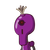# the surface area of the cube having length of one side a is = 4.9 cm is​

the surface area of the cube having length of one side a is = 4.9 cm is​

### 1 thought on “the surface area of the cube having length of one side a is = 4.9 cm is​”

1.Given : cube having length of one side 4.9 cm

To Find : The surface area of the cube

Solution:

cube having length of one side 4.9 cm

Surface area of cube = 6 ( side)²

= 6 ( 4.9)²

= 144.06  cm²

The surface area of the cube having length of one side   4.9 cm is​  144.06  cm²